Computer Graphics: the details we see every day become the wonders that tickle our fancy and lead us to strive for more realistic graphics algorithms.When constructing a world from an idea, nothing can be taken for granted.
 Home | Projects | The Raytrix | Brook | Photos | Links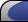What is the Raytrix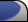The RaytrixWith Monte Carlo techniques, path tracing, and other time consuming techniques for finding rays that origin from a light source, ray tracing begs the question: how complex is it to find the destination of a ray given its source, or vice versa.Reif, Tygar and Yoshida show that it is not only time consuming to determine the destination of a ray from its input location, but that it is undecidable. They accomplish this proof by using a ray fired into a specific scene to simulate the universal Turing machine.The idea is as follows:The entire computers memory is saved as two stacks, each saved in the fraction of the ray's X and Y position respectively. If a ray were at x=1011010111.11001 y=111.1011 (in decimal x=727.78125 y=7.6875) then the X stack would have 1100100000.... and the Y stack would have 1011000000000.... on it.Given unlimited precision, this can easily hold the whole Turing machine's tape, with the head pointing to the gap between the X and the Y stacks. The stack may be manipulated as follows: There are 3 basic commands: if(top) redirect beam: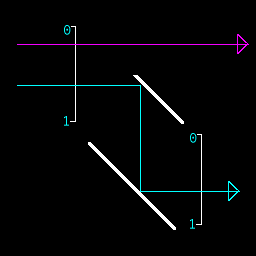add k to stack: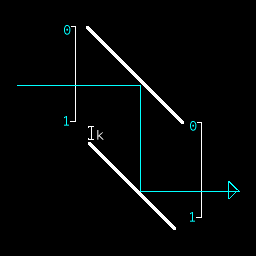push (or pop) stack: (multiply or divide ray position by 2) This functions by having 2 parabolas of different sizes which share a focus.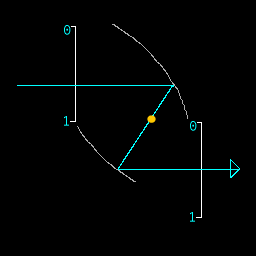Each operation may be chained by mirrors and each operation may be performed on either the X or Y position independently. Thus the machine is a Turing machine.
 Nondeterminism However, the machine has another powerful function that was not addressed in the paper: It has the ability to act as a nondeterministic Turing machine by using partially transmissive surfaces (like glass). assuming an input with the top bit off (binary representation 101110101011.011101101 first digit after . is zero) The fork device shown below can split the beam and allow two beams to continue through the entire machine.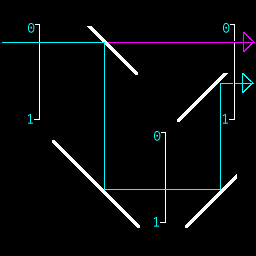The Fork Instruction operates by using a partially reflective surface to bounce the light perpendicular, then bounces it around and adds .5 on the way. That way the top most bit will end up with both zero and one. After the fork instruction another push may be executed, and so forth to fill up an arbitrary number of bits with both zero and one values. Here is an example of a number of fork commands being chained to result in 16 rays with the first four bits in both x and y being set to all possibilities.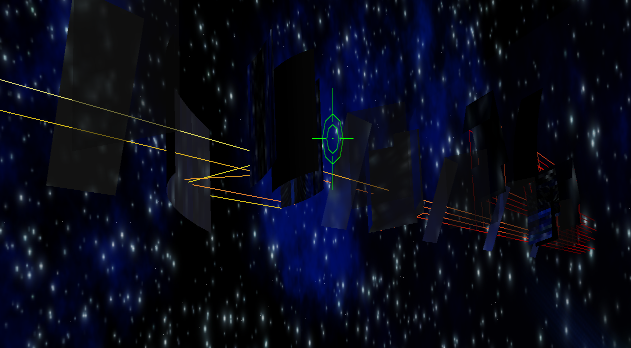(Image Height Stretched to 2x)In this way it is possible to solve problems in NP with only polynomial bounces per ray, resulting in a total polynomial number of bounces to solve the problem. So it became my goal to write a 3sat solver that used only polynomial number of bounces
 Compiler Tool-chain To write a program with the complexity of 3-sat some assistance from a compiler is in order: manually placing the mirrors one by one would be error prone and tedious. Instead I designed a compiler to do this for me. The entire tool-chain was written in the D programming language, a language still in alpha testing and undergoing a lot of flux--however also a clean high level language with careful typing rules and templates (both a requisite for a large project).I began with writing the simulator: i.e. the ray-tracer itself. The reason I could not use PBRT is that the entire memory was stored as a single number; therefore a float would not be sufficient to hold this memory--and I would have to refrain from doing approximations like square roots and actually calculate the exact answers. I started with an alpha-version D BigInteger class and built upon it a templatized Big Rational, that allowed division, mult, add, sub, and even sqrt if the fraction was square. On top of this I built a template class for vector types with the exact interface that Cg has (it turns out that D is a superset of Cg's functionality!). So I ended up using rational3 vectors for all my computation. Then I wrote a ray-tracer that could intersect rays with axis-aligned parabolas and arbitrary quads. The interface was the same as PBRT with the DifferentialGeometry class returning a normal to be reflected over and a material property BSDF. I wrote part of a uniform grid implementation, but decided it would be best to wait until things were actually going slowly than to waste time now optimizing if necessary.With the test system in place, I could fork rays, multiply them, add them, and build conditionals. I wrote little assembly statements by grouping together mirrors into 3x4x2 blocks to perform such tasks. All mirrors had closed edges, meaning that sometimes extra space was necessary to avoid edge cases failing. I ended up with 8 commands that could each produce part of a scene file:noopkill (black surface destroys ray)loop (reflect beam up and redirect to start of program)invert (flop top bit on either x or y)mod5 (set top bit to zero)agetsb (set top bit of one stack to top bit of the other)if (if the top bit of one stack is one redirect to another codepath)fork (sets top bit to both zero and one and doubles simulated # of rays)plusgets (adds a bit pattern to the stack)mulgets (divide or multiply the stack by a value)
 I combined these very low level commands into more useful commands that could be chained together by some C++ code:< var> indicates to which stack the instruction will apply < index> indicates to which bit in the stack the instruction will applyIf (< var>, < index> ); Checks if the indexth bit of stack var is set. if so execute code until EndIfIfPop (< var>, < index> ); Checks if the indexth bit of stack var is set and pops it. If so execute code until EndIfEndIf(); Loops to the beginning of the programPop(< var > ); pops value from the < var> stackPopXX(< var > , num); pops num values from < var> stackvoid PushXX(< var >, value, nbits); pushes nbits of bit pattern contained in value onto stackFork(< var >, < index> ); Sets indexth bit to both zero and oneExit(); Terminate ray with failure
 With these eight commands that were made of the previous nine, I was able to program 3-sat in assembly.Each of the instructions printed itself to a scene file with the appropriate location determined by the layout algorithm below, and each instruction also wrote itself out as a D array command, since D arrays had all the functionality of stacks. So I could test the output program as either a native x86 executable or as a raytraced (interpreted) scene file. This helped me write 3-satI had to write a layout algorithm that would lay out these carefully pieced together commands into a larger map. I accomplished this by keeping the invariant that each piece would be farther along in z, and potentially father along in x, but never decreasing in x nor increasing or decreasing in y. With this invariant, layout became trivial: I would layout the next item in z that was greatest in x and all of its children first, then I would layout the item lesser in x at the farthest unused z value. This would ensure an O(n) time layout of the building blocks of the programs. Since loops only looped to the beginning it was trivial; however, I had a mechanism for making looping constructs jump anywhere, I just did not implement it. This would have involved pushing the address of the desired instruction and then magnifying the ray until it hit the exact destination instruction location.My first successful program was the loop x=y, where x's value would be copied to y. The result of the run of the program is illustrated below: the ray is dyed by time, the darker, the more intersections it had to perform. The output is indicated with "END".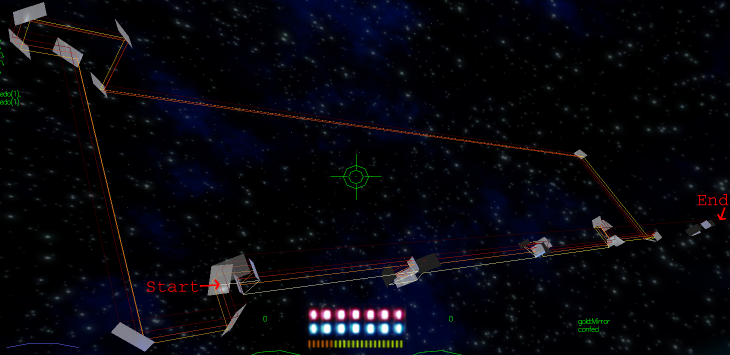It literally loops around 10 times, and it really brings to light why a loop is so named.

Finally I wrote the alleged 3-sat solver in assembly.
the input would be a bit code as follows:
you would specify the number of bits for an address of a boolean variable (say 2) and then construct a code to represent a logical boolean equation based on this.
01 would indicate the start of the sequence
00 would indicate the end of a sequence.
10 would indicate the first expression in a new triplet to be and'd with subsequent statements
111 would indicate the second expression or'd with the first
110 would indicate the third expression or'd with the second.
Then each expression would have an address (which boolean register to be tested) and a value to be tested against, either 1 or 0.
For example
echo "(r1 | r2 | r3) & (i1 | r1 | r2) & (r1 | r2 | i3) & (r0 | r0 | r0) "| ./a.out 2

Results in : Encoding 2 bits
011001010011010011010100100101001111000000000000
01: program start 10: seq start 01: look at r1 0: make it true or 10 0: make r2 true or 11 0: make r3 true. 10: AND 01 1: make r1 false or 01 0: make r1 true or 10 0: make r2 true.... ... ... ... 00: End of statement
This is fed into the following program in assembly, compiled for 2 bit addressing:
module SAT;
private import program_wrapper;
void Fail(int elementNumber) {
switch (elementNumber) {
case 0:
PushXX(X,6,3);
break;
case 1:
PushXX(X,7,3);
break;
case 2:
Exit();
}
}
void Succeed(int elementNumber,int totalbits){
switch (elementNumber) {
case 0:
PopXX(X,totalbits*2+2);

break;
case 1:
PopXX(X,totalbits+1);
break;
case 2://we achieve victory! no message to send
return;
}
}
void EvaluateTopHelper(int elementNumber, int bits, int totalbits, ulong runningSum) {
if (bits<=0) {
If(Y,runningSum);{
IfPop (X,0);
{
Fail(elementNumber);
}EndIf();
Succeed(elementNumber,totalbits);
}EndIf();
IfPop (X,0);
{
Succeed(elementNumber,totalbits);
}EndIf();
Fail(elementNumber);
}else {
IfPop(X,0);
{
EvaluateTopHelper(elementNumber,bits-1,totalbits,runningSum*2+1);
}EndIf();
EvaluateTopHelper(elementNumber,bits-1,totalbits,runningSum*2);
}
}
void EvaluateTop(int elementNumber, int bits) {
int totalbits=bits+0;
EvaluateTopHelper(elementNumber,bits,totalbits,0);
}
void SAT(int bits) {
IfPop(X,0);
{
//inner padding, after forking
IfPop(X,0);
{
//not first element
IfPop(X,0);
{
EvaluateTop(2,bits);
//we are on third and last element, don't push before looping.
}EndIf();
EvaluateTop(1,bits);//this is the second element.

}EndIf();
//this is the first element in the 3-sat
EvaluateTop(0,bits);
}EndIf();
IfPop(X,0);
{
int k=shift_left(1,bits);

for (int i=0;i< k;++i) {
Fork(Y,0);
if (i!=k-1)
PushXX(Y,0,1);
}
}EndIf();
//success! the equation is satisfiable with the following arrangement

}

 Output So After writing the program and testing the D output of the compiler in x86, I ran it through the raytracer. By now I was starting to pay dearly for my lack of acceleration structure in my ray-traced scene, but it was too late to finish off my uniform grid implementation. So I went with what I had and ran my experiments overnight. I compiled the raytrix for 1, 2 and 3 bits of addressing and tested it with both 1 and 2 bits of addressing on a number of bit equations. Sure enough if a ray didn't hit a black surface (with the Exit() command) the equation was satisfiable. Bingo: 3sat in polynomial number of bounces.Of course there's always a catch: In this case it's that the precision necessary is exponential in the size of the memory of the nondeterministic Turing machine! This means with Heisenberg uncertainty that you'd need to grow the number of atoms required to build the machine at least exponentially wrt the memory--you'd be better spending that building that many parallel computers than setting up a scene with moon-sized mirrors aligned to nanometer accuracy to solve 3sat in a polynomial number of bounces--which mind you--is not the same as polynomial time--since distance goes up exponentially :-).Alas it's still really really cool.-DanielPS: The program source may be downloaded from http://svn.dsource.org/svn/projects/deliria/turing/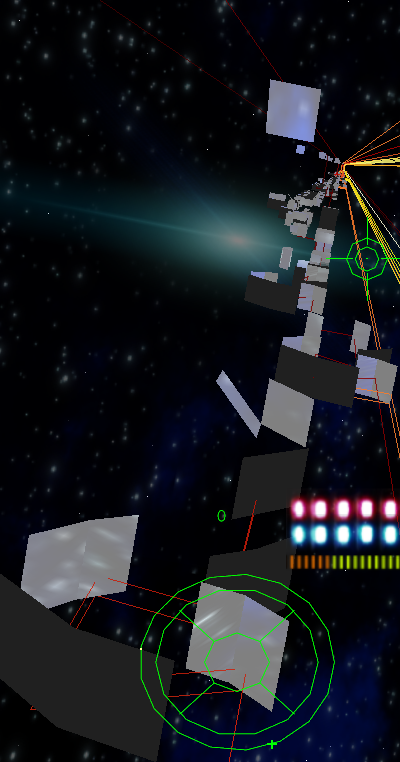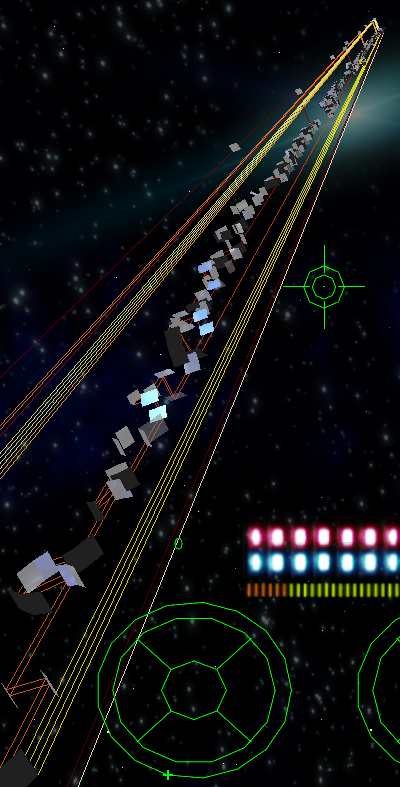John H. Reif, J. D. Tygar, and Akitoshi Yoshida. The computability and complexity of optical beam tracing. In 31st Annual Symposium on Foundations of Computer Science, volume I, pages 106-114, St. Louis, Missouri, 22-24 October 1990. IEEE.